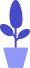# Python break continue statement usage (break out of loop)

Estimated reading: 3 minutes 64 views

We learned earlier that while loops can continue to judge until the user input is correct. But if the user does not enter the correct content, the loop will continue, and in the process of actually writing the program, we want to avoid this infinite loop from happening.

## break statement

If, as in the previous section, the program goes into an endless loop, we have a shortcut to make the while loop break immediately. This is the break statement.

Take the code just now as an example, we slightly modify it, the code is as follows.

``````name=""
while name!="Johnson":
print("Please input a name. Enter 'q' to quit: ")
name=input()
if name == "q":
break``````

This time we have left a “back door”, if the user can’t enter the name correctly, he can enter the letter “q” to exit the loop, as shown in Figure 1.

figure 1
We can add a break statement somewhere in the program to make sure the user doesn’t get stuck in a program that never exits. For example, in Python, we often use while True such a seemingly permanent loop statement, but at the same time add a break conditional judgment in the code to terminate the loop when a condition inside the loop is met.

Let’s look at an example. Suppose the password of a safe is “338822”. The program requires the user to enter the correct password before the safe can be opened. The code is as follows.

``````password = "338822"
while True:
userInput = input("Please enter a 6-digit password: ")
if userInput == password:
print("Open the safe")
break
else:
print("The password you entered is incorrect, please try again")``````

Because the while is followed by True, it means that the entire loop will be executed all the time, and the loop will not jump out until the input number is equal to the set password, as shown in Figure 2.

Figure 2

## continue statement

In a while loop, if we just want to return to the beginning of the loop, and then decide whether to continue the loop based on a condition, instead of exiting the loop directly, we can use the continue statement. For example, we want to print out numbers from 1 to 10, but not multiples of 3, the code is as follows.

``````number=0
while number<10:
number=number+1
if number %3 ==0:
continue
print ("The current number is :"+str(number))``````

We first create a variable called number and set its initial value to 0. When starting the while loop, first check if number is less than 10. Since the value of number is now 0, which is less than 10, the statements in the code block (the body of the loop) are executed. First, the statement number=number+1 will increment the value of number by 1.

Next, the statement number %3 ==0 checks whether number is divisible by 3. If this condition is True, execute the continue statement to jump out of this loop and directly enter the next loop; otherwise, print the value of the current number. The result of running the program is shown in Figure 3.

Image 3